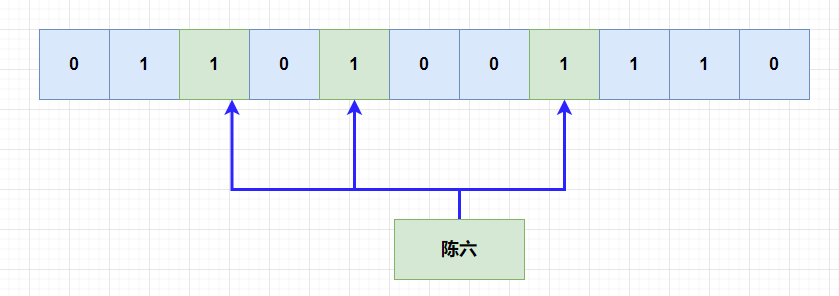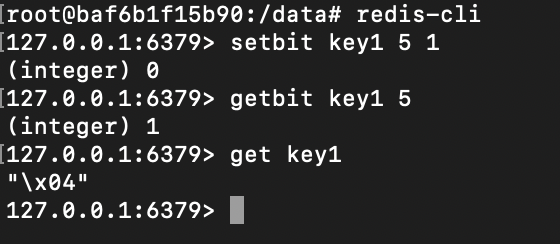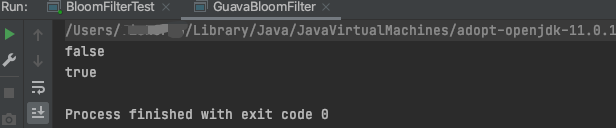• 累计撰写 141 篇文章
• 累计创建 37 个标签
• 累计收到 11 条评论

【实战问题】-- 布隆过滤器的三种实践：手写，Redission以及Guava（2）2021-05-13 / 0 评论 / 0 点赞 / 315 阅读 / 0 字

布隆过滤器手写布隆过滤器

BitSet bits = new BitSet(size);

• 清空位数组的数据
• 翻转某一位的数据
• 设置某一位的数据
• 获取某一位的数据
• 获取当前的bitSet的位数

• 位数组的大小空间，需要指定，其他相同的时候，位数组的大小越大，hash冲突的可能性越小。
• 多个hash函数，我们需要使用hash数组来存，hash函数需要如何设置呢？为了避免冲突，我们应该使用多个不同的质数来当种子。
• 方法：主要实现两个方法，一个往布隆过滤器里面添加元素，另一个是判断布隆过滤器是否包含某个元素。

import java.util.BitSet;

public class MyBloomFilter {

// 默认大小
private static final int DEFAULT_SIZE = Integer.MAX_VALUE;

// 最小的大小
private static final int MIN_SIZE = 1000;

// 大小为默认大小
private int SIZE = DEFAULT_SIZE;

// hash函数的种子因子
private static final int[] HASH_SEEDS = new int[]{3, 5, 7, 11, 13, 17, 19, 23, 29, 31};

// 位数组，0/1,表示特征
private BitSet bitSet = null;

// hash函数
private HashFunction[] hashFunctions = new HashFunction[HASH_SEEDS.length];

// 无参数初始化
public MyBloomFilter() {
// 按照默认大小
init();
}

// 带参数初始化
public MyBloomFilter(int size) {
// 大小初始化小于最小的大小
if (size >= MIN_SIZE) {
SIZE = size;
}
init();
}

private void init() {
// 初始化位大小
bitSet = new BitSet(SIZE);
// 初始化hash函数
for (int i = 0; i < HASH_SEEDS.length; i++) {
hashFunctions[i] = new HashFunction(SIZE, HASH_SEEDS[i]);
}
}

// 添加元素，相当于把元素的特征添加到位数组
for (HashFunction f : hashFunctions) {
// 将hash计算出来的位置为true
bitSet.set(f.hash(value), true);
}
}

// 判断元素的特征是否存在于位数组
public boolean contains(Object value) {
boolean result = true;
for (HashFunction f : hashFunctions) {
result = result && bitSet.get(f.hash(value));
// hash函数只要有一个计算出为false，则直接返回
if (!result) {
return result;
}
}
return result;
}

// hash函数
public static class HashFunction {
// 位数组大小
private int size;
// hash种子
private int seed;

public HashFunction(int size, int seed) {
this.size = size;
this.seed = seed;
}

// hash函数
public int hash(Object value) {
if (value == null) {
return 0;
} else {
// hash值
int hash1 = value.hashCode();
// 高位的hash值
int hash2 = hash1 >>> 16;
// 合并hash值(相当于把高低位的特征结合)
int combine = hash1 ^ hash1;
// 相乘再取余
return Math.abs(combine * seed) % size;
}
}

}

public static void main(String[] args) {
Integer num1 = new Integer(12321);
Integer num2 = new Integer(12345);
MyBloomFilter myBloomFilter =new MyBloomFilter();
System.out.println(myBloomFilter.contains(num1));
System.out.println(myBloomFilter.contains(num2));

System.out.println(myBloomFilter.contains(num1));
System.out.println(myBloomFilter.contains(num2));

}
}

false
false
true
true

// 带参数初始化
public BloomFilter(int num,double rate) {
// 计算位数组的大小
this.size = (int) (-num * Math.log(rate) / Math.pow(Math.log(2), 2));
// hsah 函数个数
this.hashSize = (int) (this.size * Math.log(2) / num);
// 初始化位数组
this.bitSet = new BitSet(size);
}

Redis实现

setbit key offset valuegitbit key offset

<dependencies>
<dependency>
<groupId>org.redisson</groupId>
<artifactId>redisson</artifactId>
<version>3.11.2</version>
</dependency>
</dependencies>

docker run -d --name redis -p 6379:6379 redis --requirepass "password"

import org.redisson.Redisson;
import org.redisson.api.RBloomFilter;
import org.redisson.api.RedissonClient;
import org.redisson.config.Config;

public class BloomFilterTest {
public static void main(String[] args) {
Config config = new Config();
// 相当于创建了redis的连接
RedissonClient redisson = Redisson.create(config);

RBloomFilter<String> bloomFilter = redisson.getBloomFilter("myBloomFilter");
//初始化,预计元素数量为100000000,期待的误差率为4%
bloomFilter.tryInit(100000000,0.04);
//将号码10086插入到布隆过滤器中

System.out.println(bloomFilter.contains("123456"));//false
System.out.println(bloomFilter.contains("12345"));//true
}
}<dependency>
<artifactId>guava</artifactId>
<version>18.0</version>
</dependency>

public class GuavaBloomFilter {
public static void main(String[] args) {
BloomFilter<String> bloomFilter = BloomFilter.create(
Funnels.stringFunnel(Charsets.UTF_8),1000000,0.04);

bloomFilter.put("Sam");

System.out.println(bloomFilter.mightContain("Jane"));
System.out.println(bloomFilter.mightContain("Sam"));
}
}2020年我写了什么？

0